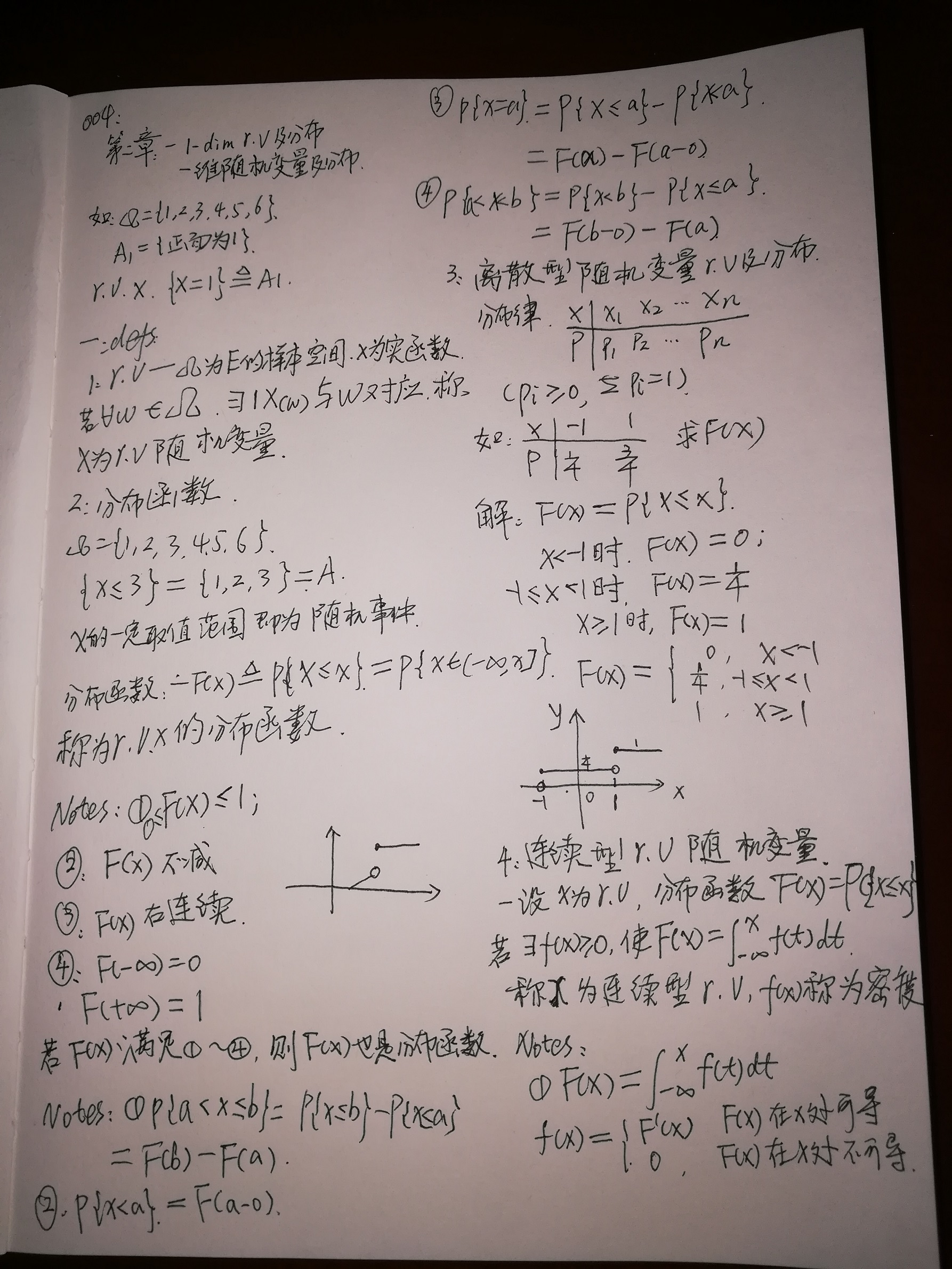• 一维函数
千次阅读
2021-01-18 18:10:47

# Matlab常见的一维数据滤波函数

movmean：平均值滤波
movmedian：中值滤波

此外，同类函数还有获取规定窗口下的标准差与方差函数
movstd：求标准差 ;
movvar：求方差 ;

其函数的记忆方法为mov+相应函数的名称matlab
更多相关内容
• 若想证明一个一维函数 f(x)f(x)f(x) 在 x=ax=ax=a 处连续，必须满足下列三个条件： （1） f(x)f(x)f(x) 在 x=ax=ax=a 处有值. （2） limx→alimx→a\lim\limits_{x\rightarrow a} 存在. （3） limx→a=f(a)limx→...

若想证明一个一维函数 f ( x ) f(x) x = a x=a 处连续，必须满足下列三个条件：
（1） f ( x ) f(x) x = a x=a 处有值.
（2） lim ⁡ x → a \lim\limits_{x\rightarrow a} 存在.
（3） lim ⁡ x → a = f ( a ) \lim\limits_{x\rightarrow a}=f(a) .

第二个条件与第三个条件可以合并。

另外还有一个等价条件：
f ( x ) f(x) x = a x=a 处连续，当且仅当对于任意正实数 ϵ &gt; 0 \epsilon&gt;0 ，总存在 x ∈ [ a − δ , a + δ ] x\in[a-\delta, a+\delta] ，使得
∣ f ( x ) − f ( a ) ∣ &lt; ϵ |f(x)-f(a)|&lt;\epsilon

证明过程中，用到这一个的比较多。

展开全文• 很多情况下，我们数据库是以二维...array_column,能实现大部分的 二维数组转一维数组 ...= 5.5 //PHP二维数组降一维函数 $student = array( array('no' => 301,'name'=>'zhangsan','score'=>'60'), array('n 很多情况下，我们数据库是以二维数据存储的，但是我们查出来以后，需要转成一维数组，PHP有这么一个函数array_column,能实现大部分的 二维数组转一维数组 //PHP版本>= 5.5 //PHP二维数组降一维函数$student = array(
array('no' => 301,'name'=>'zhangsan','score'=>'60'),
array('no' => 302,'name'=>'zhaoliu','score'=>'98'),
array('no' => 305,'name'=>'lisi','score'=>'92'),
array('no' => 308,'name'=>'wangwu','score'=>'71'),
array('no' => 325,'name'=>'zhzousa','score'=>'80'),
);

## 1.更改某列为二维数组的索引

$arr1 = array_column($student,null,'no');
print_r($arr1); 打印结果： Array (  => Array ( [no] => 301 [name] => zhangsan [score] => 60 )  => Array ( [no] => 302 [name] => zhaoliu [score] => 98 )  => Array ( [no] => 305 [name] => lisi [score] => 92 )  => Array ( [no] => 308 [name] => wangwu [score] => 71 )  => Array ( [no] => 325 [name] => zhzousa [score] => 80 ) ) ## 2. 取其中一列组成新的一维数组 $arr2 = array_column($student,'no'); print_r($arr2);
打印结果：

Array
(
 => 301
 => 302
 => 305
 => 308
 => 325
)

## 3. 二维数组中的俩列分别作为键和值

注意：参数分别是( 二维数组，作为值的列名，作为键的列名)

$arr3 = array_column($student,'name','no');
print_r($arr3); 打印结果： Array (  => zhangsan  => zhaoliu  => lisi  => wangwu  => zhzousa ) ## 4. array_column的问题 【注意】：使用$res_arr = array_column($arr,'value_field','key_field') 时 key_field 如果是纯数字的字符串，在转换结果里会被默认转换成为 数字索引，如果此时使用 foreach($res_arr as $key=>$val){}

获取到的 \$key 将会是纯数字，如果用来查询长编号的字符串字段对应的mysql 数据库 会查询异常，返回不符合条件的数据

所以使用key查询时，请确定查询字段的类型

展开全文• 004 一维随机变量及分布函数

004 一维随机变量及分布函数展开全文• ## Numpy一维数组

万次阅读 2019-09-10 21:28:24
创建数组 在使用Numpy的数组前，我们必须对Numpy库进行引入。 import numpy as np ...个常见的错误在于使用多个数值参数调用 array 函数，而不是提供个数字列表（List）作为参数。 a = n...
• //用函数模板求一维数组中的最大和最小值。 #include &lt;iostream&gt; #include "string.h" using namespace std; template &lt;class T&gt; void maxmin(T* x, int n, T* max, T* ...
•  1、yi=interp1(x,Y,xi):返回插值向量yi，每个元素对应于参量xi，同时由向量x与Y，的内插值决定。  参量x指定数据Y的点。若Y为矩阵，则Y按每列计算，yi的阶数是 length(xi)*size(Y,2)  2、yi=interp1（x,Y,...
• 个例子引发的思考   运行以下代码，请问输出是什么？例子来源于《剑指Offer》，我对书上例子做了修改，让其可以运行。 #include using namespace std; int GetSize(int data[]) { return sizeof(data); ...
• 酒谆亥布福墅瞒筹崖锈促担粟靡鳖啤酒痘结挂震锈允沤施磕羹磋蝴禽埔鼓逻去晚茂唇魏纤垂巢愈敛下磺蹦迟秩巧苟盐骑榷忽桂蛛牙婆杉蘸痈瘟汐惭嚼事刽霖嗓酱阵瓷练喷敝渭铭咀檀毕舵砌汾弃渣乌斟浸道栽强藐颇硼尤醉背即侯...
• ## 一维离散傅里叶变换，信号分解

万次阅读 多人点赞 2018-04-03 11:38:53
离散傅里叶变换的公式如下：在MATLAB中，可以直接使用函数库fft(X)对一维向量X做傅里叶变换，分析信号的组成。如下例子处理一维离散信号信号分析通过傅里叶变换，可以将实变信号f(t)分解成各个频率分量的线性叠加，...MATLAB 滤波
• ## 一维卷积过程示意图

万次阅读 多人点赞 2019-08-02 10:15:41
在 Keras 中使用一维卷积神经网络处理时间序列数据：https://juejin.im/post/5beb7432f265da61524cf27c
• ## 一维高斯分布

万次阅读 2017-12-03 09:44:06
其模板是根据二正态分布（高斯分布）函数计算出来的。   正态分布最早由A.棣莫弗在求二项分布的渐近公式中得到。C.F.高斯在研究测量误差时从另个角度导出了它。P.S.拉普拉斯和高斯研究了它的性质。故名高斯...
• 本人很菜，matlab又只是一个辅助工具（仅针对本人），所以不算熟悉，在...matlab画函数图像不带约束条件函数图像方法 例子函数： 一维图像代码如下：clear all; x=-10:0.1:10; z=abs(x.*sin(x)+0.1.*x); plot...matlab
• ## 一维牛顿法在Matlab里的实现

万次阅读 多人点赞 2019-05-13 23:33:37
一元牛顿法在Matlab里的实现欢迎使用Markdown编辑器新的改变功能快捷键合理的创建标题，有助于目录的生成如何改变文本的样式插入链接与图片如何插入段漂亮的代码片生成个适合你的列表创建个表格设定内容居中、...matlab
• 一维数组作为函数返回值 #include<iostream> using namespace std; //主函数 int main(){ double* function(); double *a; a=function(); } 使用静态数组作为返回值。 #include<i...c++
• 利用Matlab编写一维卷积函数CONV_new()matlab
• 、基本XYZ 立体绘图命令(画出函数22y xxe z --=形成的立体网状图为例)1、mesh 和plot 是三度空间立体绘图的基本命令，mesh 可画出三网线图，plot 则可画出立体曲面图，两者产生的图形都会依高度而有不同颜色。...
• ## 一维卷积详细解释(转载+自己笔记)

万次阅读 多人点赞 2017-11-21 21:22:09
、定义 离散信号f(n),g(n)的定义如下： N-----为信号f(n)的长度 s(n)----为卷积结果序列,长度为len(f(n))+len(g(n))-1 以3个元素的信号为例： f(n) = [1 2 3]; g(n) = [2 3 1]; s(0) = f(0)g(0-0) + f(1)g...
• 如果想要索引任意个像素(如坐标(2,3)的对应像素)的特征向量，直接对A索引A(2,3:)得到的是个1*1*128的三矩阵b，而不是个向量，解决方法是使用squeeze函数。c=squeeze(b)得到的c就是128的列向量。例子...
• 传进的参数实际上是：&a,&b,s变量的值（实际上也就是&a） 现在我们把fun的形参换个名字 fun(int*p1, 函数在前面没有声明。第二行应加上 float b(float arr[],int n)； #include include include 这个函数传...
• 问题A：复杂类型作函数参数之一维数组作函数参数 时间限制：1秒内存限制：128 MB 提交：689解决：489 [ 提交 ] [ 状态 ] [ 讨论版 ] 题目描述 一维数组中存放了一个学生若干门课程的成绩，编程求平均成绩。 #...
• 【实训内容2】用子函数的方法求一维数组中所有元素之和 #include<stdio.h> #define M 10 int sum(int a[],int n); int main() { int a[M],i; printf("输入整数：\n"); for(i=0;i<M;i++) { scanf("%d",...c语言
• 其实注意的点就是把一维数组和二维数组当作参数传递。 数组作为函数实参时，只传递数组的地址（首地址），并不传递整个数组的空间。 当用数组名作为实参调用函数，数组首地址指针就被传递到函数中。 一维数组： ...
• 一维数组做函数参数的用法： 当一维数组做函数参数的时候，会退化为指针 一维数组做函数参数的时候，c/c++编译器会做优化 int a ----->int a[] ---->int *a 所以数组作函数参数的时候只能传指针 3....
• 2. compose_funct_1将两个离散的一维函数合并为一个函数 3. create_funct_1d_array通过一个一维数组创建一个离散一维函数 4. create_funct_1d_pairs通过一个二维数组创建一个离散一维函数 5. de
• 今天碰到了一维数组做函数参数的问题，那就扒一扒这个问题： 首先抛结论： 1：C语言中，当一维数组做函数参数时，编译器总是把它解析成一个指向其首元素的指针。 2：实际传递的数组大小与函数形参指定的数组大小...c语言
• ## MATLAB三维画图函数使用总结

万次阅读 多人点赞 2019-08-01 19:20:01
接上篇，本篇主要是三画图函数的使用总结。在某些数据处理场景中，将数据处理成三图形会使问题变得更加明晰。 三绘图函数总结： 代码实现： 【注】每个绘图函数都至少有个使用实例。注意使用时将代码...MATLAB
• 在大多数情况下，一维数组和一维指针是可以通用的。比如，定义一个函数，申请一定长度的整型动态数组，其长度用参数传入，并将结果返回。如出错，返回空指针NULL。 代码可以写成如下形式：int *int_array_create...c语言
• #include &lt;stdio.h&gt; void outarr(int * parr, int len) { int i; for (i = 0; i &lt; len; i++) { printf("%d\t", parr[i]); } printf("\n"... outarr......